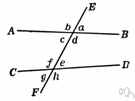# exterior angle

Also found in: Thesaurus, Encyclopedia, Wikipedia.

## exterior angle

n.
1. The angle between any side of a polygon and an extended adjacent side.
2. Any of the four angles that do not include a region of the space between two lines intersected by a transversal.

## exterior angle

n
1. (Mathematics) an angle of a polygon contained between one side extended and the adjacent side
2. (Mathematics) any of the four angles made by a transversal that are outside the region between the two intersected lines
Collins English Dictionary – Complete and Unabridged, 12th Edition 2014 © HarperCollins Publishers 1991, 1994, 1998, 2000, 2003, 2006, 2007, 2009, 2011, 2014

## exte′rior an′gle

n.
1. an angle formed outside parallel lines by a third line that intersects them.
2. an angle formed outside a polygon by one side and an extension of an adjacent side; the supplement of an interior angle of the polygon.
[1885–90]
exterior angles
Angles 1, 2, 7, and 8 are exterior angles. Angles 1 and 8 and angles 2 and 7 are alternate exterior angles.

## ex·te·ri·or angle

(ĭk-stîr′ē-ər)
The angle formed between a side of a polygon and an extended adjacent side. Compare interior angle.
ThesaurusAntonymsRelated WordsSynonymsLegend:
 Noun 1exterior angle - the supplement of an interior angle of a polygonexternal angleangle - the space between two lines or planes that intersect; the inclination of one line to another; measured in degrees or radianship - (architecture) the exterior angle formed by the junction of a sloping side and a sloping end of a roof
Based on WordNet 3.0, Farlex clipart collection. © 2003-2012 Princeton University, Farlex Inc.
Translations

## exterior angle

n (Math) → Außenwinkel m
Collins German Dictionary – Complete and Unabridged 7th Edition 2005. © William Collins Sons & Co. Ltd. 1980 © HarperCollins Publishers 1991, 1997, 1999, 2004, 2005, 2007
References in periodicals archive ?
The purpose here is to let students see that half of [angle]DOA is an exterior angle of the triangle and equal to [angle]OCA.
###(vi)###An exterior angle of a triangle is greater in measure than###No
The angle with any other vertex which lies on this line (for example, angle A[C.sub.1]B) will be less than angle ACB because [angle]A[C.sub.1]B < [angle]A[A.sub.1]B (the exterior angle A[A.sub.1]B of the triangle B[A.sub.1][C.sub.1] is bigger than the remote interior angle [angle]A[C.sub.1]B) and [angle]A[A.sub.1]B = [angle]ACB (inscribed angles).
Initially, they defined it as a figure made with two half lines, but they were not satisfied with this definition because it was not clear whether the definition referred to interior angle or exterior angle (see Figure 2).
A regular polygon has an exterior angle of 20 degrees.
Photography for the marketing brochure was not simple as no exterior angle gives any clue to the wide open spaces inside.

Site: Follow: Share:
Open / Close